### Similar Triangles Class 10th Mathematics AP Board Solution

##### Question 1.Prove that the sum of the squares of the sides of a rhombus is equal to the sum of the squares of its diagonals.Answer:Need to prove that the sum of the squares of the sides of a rhombus is equal to the sum of the squares of its diagonals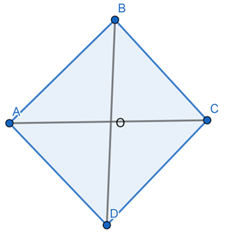ABCD is a rhombus in which diagonals AC and BD intersect at point O.We need to prove AB2 + BC2 + CD2 + DA2 = AC2 + DB2⇒ In Δ AOB; AB2 = AO2 + BO2⇒ In Δ BOC; BC2 = CO2 + BO2⇒ In Δ COD; CD2 = DO2 + CO2⇒ In Δ AOD; AD2 = DO2 + AO2⇒ Adding the above 4 equations we get⇒ AB2 + BC2 + CD2 + DA2 = AO2 + BO2 + CO2 + BO2 + DO2 + CO2 + DO2 + AO2⇒ = 2(AO2 + BO2 + CO2 + DO2)Since, AO2 = CO2 and BO2 = DO2= 2(2 AO2 + 2 BO2)= 4(AO2 + BO2) ……eq(1)Now, let us take the sum of squares of diagonals⇒ AC2 + DB2 = (AO + CO)2 + (DO+ BO)2= (2AO)2 + (2DO)2= 4 AO2 + 4 BO2 ……eq(2)From eq(1) and eq(2) we get⇒ AB2 + BC2 + CD2 + DA2 = AC2 + DB2Hence, provedQuestion 2.ABC is a right triangle right angled at B. Let D and E be any points on AB and BC respectively.Prove that AE2 + CD2 = AC2 + DE2 .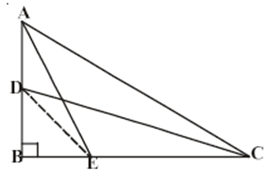Answer:Given, ABC as a right angled triangleNeed to prove that AE2 + CD2 = AC2 + DE2⇒ In right angled triangle ABC and DBC, we have⇒ AE2 = AB2 + BE2 ………..eq(1)⇒ DC2 = DB2 + BC2 ……….eq(2)⇒ Adding equation 1 and 2 we have⇒ AE2 + DC2 = AB2 + BE2 + DB2 + BC2= (AB2 + BC2) + (BE2 + DB2)⇒ Since AB2 + BC2 = AC2 in right angled triangle ABC∴ AC2 + DE2Hence provedQuestion 3.Prove that three times the square of any side of an equilateral triangle is equal to four times the square of the altitude.Answer: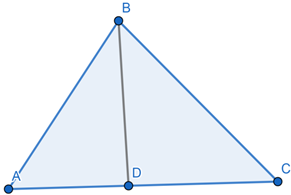Given, an equilateral triangle ABC, in which AD perpendicular BCNeed to prove that 3 AB2 = 4AD2⇒ Let AB = BC = CA = a⇒ In Δ ABD and Δ ACD⇒ AB = AC, AD = AD and ∠ ADB = ∠ ADC∴ Δ ABD ≅ Δ ACD∴ BD = CD =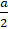⇒ Now, in Δ ABD, ∠ D = 90°∴ AB2 = BD2 + AD2⇒ AB2 =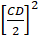+ AD2=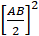+ AD23AB2 = 4 AD2Question 4.PQR is a triangle right angled at P and M is a point on QR such that PM ⊥ QR. Show that PM2 = QM.MR.Answer: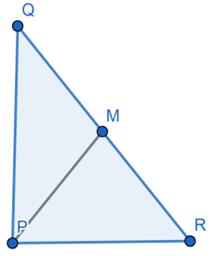⇒ Let ∠MPR = x⇒ In Δ MPR, ∠MRP = 180-90-x⇒ ∠MRP = 90-xSimilarly in Δ MPQ,∠MPQ = 90-∠MPR = 90-x⇒ ∠MQP = 180-90-(90-x)⇒ ∠MQP = xIn Δ QMP and Δ PMR⇒ ∠MPQ = ∠MRP⇒ ∠PMQ = ∠RMP⇒ ∠MQP = ∠MPR⇒ Δ QMP ∼ Δ PMR⇒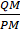=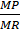⇒ PM2 = MR × QMHence provedQuestion 5.ABD is a triangle right angled at A and AC ⊥ BDShow thati. AB2 = BC . BDii. AC2 = BC . DCiii. AD2 = BD . CD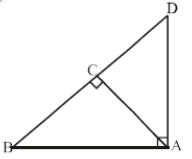Answer:Given, ABCD is a right angled triangle and AC is perpendicular to BD(i) consider two triangles ACB and DAB⇒ We have ∠ ABC = ∠ DBC⇒ ∠ ACB = ∠ DAB⇒ ∠ CAB = ∠ ADB∴ they are similar and corresponding sides must be proportionali.e, ∠ ADC = ∠ ADB⇒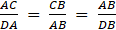∴ AB2 = BC × CD(ii) ∠ BDA = ∠ BDC = 90°⇒ ∠ 3 = ∠ 2 = 90° ∠ 1⇒ ∠ 2 + ∠ 4 = 90° ∠ 2⇒ From AAA criterion of similarity we have in two triangles if the angles are equal, then sides opposite to the equal angles are in the same ratio (or proportional) and hence the triangles are similarTheir corresponding sides must be proportional⇒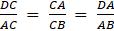⇒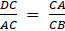⇒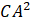= BC × DC(iii) In two triangles ADB and ABC we have∠ ADC = ∠ ADB⇒ ∠ DCA = ∠ DAB⇒ ∠ DAC = ∠ DBA⇒ ∠ DCA = ∠ DAB⇒ Triangle ADB and ABC are similar and so their corresponding sides must be proportion.⇒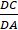=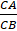=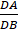⇒=⇒ AD2 = DB × DCHence provedQuestion 6.ABC is an isosceles triangle right angled at C. Prove that AB2 = 2AC2.Answer:Since the triangle is right angled at C∴ the side AB is hypotenuse.⇒ Let the base of the triangle be AC and the altitude be BC.⇒ Applying the Pythagorean theorem⇒ HYP2 = Base2 + Alt2⇒ AB2 = AC2 + BC2Since the triangle is isosceles triangle two of the sides shall be equal∴ AC = BCThus AB2 = AC2 + BC2AB2 = 2AC2Hence, provedQuestion 7.‘O’ is any point in the interior of a triangle ABC.OD ⊥ BC, OE ⊥ AC and OF ⊥ AB, show thati. OA2 + OB2 + OC2 – OD2 – OE2 – OF2 = AF2 + BD2 + CE2ii. AF2 + BD2 + CE2 = AE2 + CD2 + BF2.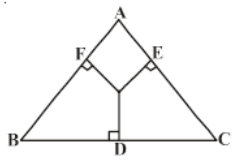Answer:Given, Δ ABC, OD ⊥ BC, OE ⊥ AC and OF ⊥ AB,Need to prove OA2 + OB2 + OC2-OD2-OE2-OF2 = AF2 + BD2 + CE2⇒ Join point O to A,B and C(i) ∠AFO = 90°AO2 = AF2 + OF2⇒ AF2 = AO2 - OF2 …….eq(1)Similarly BD2 = BO2-OD2 …..eq(2)⇒ CE2 = CO2-OE2 …..eq(3)Adding eq(1), (2) and (3) we get⇒ AF2 + BD2 + CE2 = OA2 + OB2 + OC2-OD2-OE2-OF2(ii) AF2 + BD2 + CE2 = (AO2-OE2) + ( BO2-OF2) + ( CO2-OD2)= AE2 + CD2 + BF2Hence, provedQuestion 8.A wire attached to vertically pole of height 18m is24m long and has a stake attached to other end. How far from the base of the pole should the stake be driven so that the wire will be taut?Answer:Given, height of a pole is 18 and wire attached is 24mNeed to find the distance from the base to keep wire taut⇒ Let AB be a wire and pole be BC⇒ to keep the wire taut let it be fixed at A⇒ AB2 = AC2 + BC2⇒ 242 = AC2 + 182⇒ AC2 = 242 + 182⇒ AC2 = 576-324⇒ = 252⇒ AC = √ 252= √(36 × 7)= 6√ 7Hence, the stake may be placed at a distance of 6√ 7m the base of poleQuestion 9.Two poles of heights 6m and 11m stand on a plane ground. If the distance between the feet of the pole is 12m find the distance between their tops.Answer: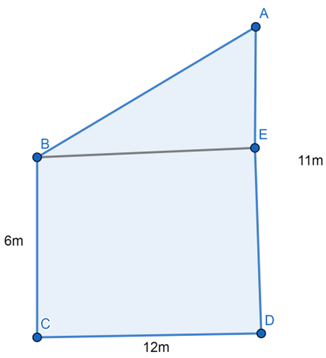Given, BC = 6m, AD = 11m, BC = EDAnd AE = AD-ED = 11-6 = 5mBE = CD = 12mNeed to find AB⇒ Now, In Δ ABE, ∠E = 90°⇒ AB2 = AE2 + BE2⇒ AB2 = 52 + 122 = 169⇒ AB2 = 169⇒ AB = 13mThe distance between their tops is 13mQuestion 10.In an equilateral triangle ABC, D is on a side BC such that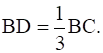Prove that 9AD2 = 7AB2.Answer: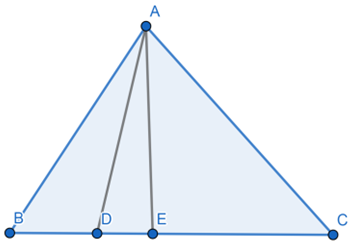Given, ABC is a equilateral triangle where AB = BC = AC and BD =BCDraw AE perpendicular BC⇒ Δ ABE ≅ Δ ACE∴ BE = EC =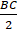⇒ Now in Δ ABE, AB2 = BE2 + AE2⇒ also AD2 = AE2 + DE2∴ AB2 –AD2 = BE2 – DE2= BE2 – (BE-BD)2= ()2 –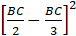= (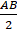)2 –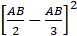AB2-AD2 = 2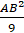Or 7 AB2 = 9 AD2Hence, provedQuestion 11.In the given figure, ABC is a triangle right angled at B. D and E are points on BC trisect it.Prove that 8AE2 = 3AC2 + 5AD2.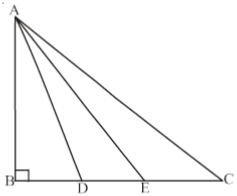Answer:Given, ABC triangleNeed to prove 8AE2 = 3AC2 + 5AD2⇒ In Δ ABD, ∠B = 90°∴ AC2 = AB2 + BC2 ….eq(1)⇒ Similarly, AE2 = AB2 + BE2 …….eq(2)⇒ And AD2 = AB2 + BD2 …….eq(3)⇒ Form eq(1)⇒ 3AC2 = 3AB2 + 3 BC2 …eq(4)⇒ From eq(2)⇒ 5AD2 = 5AB2 + 5BD2 ……eq(5)Adding equation (4) and (5)3AC2 + 5AD2 = 8AB2 + 3 BC2 + 5BD2= 8AB2 + 3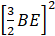+ 5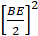= 8(AB2 + BE2)= 8AE2Hence, provedQuestion 12.ABC is an isosceles triangle right angled at B. Similar triangles ACD and ABE are constructed on sides AC and AB. Find the ratio between the areas of ΔABE and ΔACD.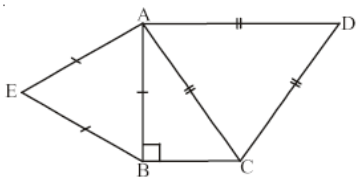Answer:Given, ABC is an isosceles triangle in which ∠B = 90°Need to find the ratio between the areas of Δ ABE and Δ ACD⇒ AB = BC⇒ By Pythagoras theorem, we have AC2 = AB2 + BC2⇒ since AB = BC⇒ AC2 = AB2 + AB2⇒ AC2 = 2 AB2 …..eq(1)⇒ it is also given that Δ ABE ∼ Δ ACD(ratio of areas of similar triangles is equal to ratio of squares of their corresponding sides)⇒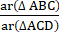=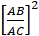⇒=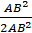from 1⇒=∴ ar(Δ ABC):ar(Δ ACD) = 1:2Hence the ratio is 1:2

PDF FILE TO YOUR EMAIL IMMEDIATELY PURCHASE NOTES & PAPER SOLUTION. @ Rs. 50/- each (GST extra)

HINDI ENTIRE PAPER SOLUTION

MARATHI PAPER SOLUTION

SSC MATHS I PAPER SOLUTION

SSC MATHS II PAPER SOLUTION

SSC SCIENCE I PAPER SOLUTION

SSC SCIENCE II PAPER SOLUTION

SSC ENGLISH PAPER SOLUTION

SSC & HSC ENGLISH WRITING SKILL

HSC ACCOUNTS NOTES

HSC OCM NOTES

HSC ECONOMICS NOTES

HSC SECRETARIAL PRACTICE NOTES

# 2019 Board Paper Solution

HSC ENGLISH SET A 2019 21st February, 2019

HSC ENGLISH SET B 2019 21st February, 2019

HSC ENGLISH SET C 2019 21st February, 2019

HSC ENGLISH SET D 2019 21st February, 2019

SECRETARIAL PRACTICE (S.P) 2019 25th February, 2019

HSC XII PHYSICS 2019 25th February, 2019

CHEMISTRY XII HSC SOLUTION 27th, February, 2019

OCM PAPER SOLUTION 2019 27th, February, 2019

HSC MATHS PAPER SOLUTION COMMERCE, 2nd March, 2019

HSC MATHS PAPER SOLUTION SCIENCE 2nd, March, 2019

SSC ENGLISH STD 10 5TH MARCH, 2019.

HSC XII ACCOUNTS 2019 6th March, 2019

HSC XII BIOLOGY 2019 6TH March, 2019

HSC XII ECONOMICS 9Th March 2019

SSC Maths I March 2019 Solution 10th Standard11th, March, 2019

SSC MATHS II MARCH 2019 SOLUTION 10TH STD.13th March, 2019

SSC SCIENCE I MARCH 2019 SOLUTION 10TH STD. 15th March, 2019.

SSC SCIENCE II MARCH 2019 SOLUTION 10TH STD. 18th March, 2019.

SSC SOCIAL SCIENCE I MARCH 2019 SOLUTION20th March, 2019

SSC SOCIAL SCIENCE II MARCH 2019 SOLUTION, 22nd March, 2019

XII CBSE - BOARD - MARCH - 2019 ENGLISH - QP + SOLUTIONS, 2nd March, 2019

# HSCMaharashtraBoardPapers2020

(Std 12th English Medium)

HSC ECONOMICS MARCH 2020

HSC OCM MARCH 2020

HSC ACCOUNTS MARCH 2020

HSC S.P. MARCH 2020

HSC ENGLISH MARCH 2020

HSC HINDI MARCH 2020

HSC MARATHI MARCH 2020

HSC MATHS MARCH 2020

# SSCMaharashtraBoardPapers2020

(Std 10th English Medium)

English MARCH 2020

HindI MARCH 2020

Hindi (Composite) MARCH 2020

Marathi MARCH 2020

Mathematics (Paper 1) MARCH 2020

Mathematics (Paper 2) MARCH 2020

Sanskrit MARCH 2020

Sanskrit (Composite) MARCH 2020

Science (Paper 1) MARCH 2020

Science (Paper 2)

Geography Model Set 1 2020-2021

MUST REMEMBER THINGS on the day of Exam

Are you prepared? for English Grammar in Board Exam.

Paper Presentation In Board Exam

How to Score Good Marks in SSC Board Exams

Tips To Score More Than 90% Marks In 12th Board Exam

How to write English exams?

How to prepare for board exam when less time is left

How to memorise what you learn for board exam

No. 1 Simple Hack, you can try out, in preparing for Board Exam

How to Study for CBSE Class 10 Board Exams Subject Wise Tips?

JEE Main 2020 Registration Process – Exam Pattern & Important Dates

NEET UG 2020 Registration Process Exam Pattern & Important Dates

How can One Prepare for two Competitive Exams at the same time?

8 Proven Tips to Handle Anxiety before Exams!